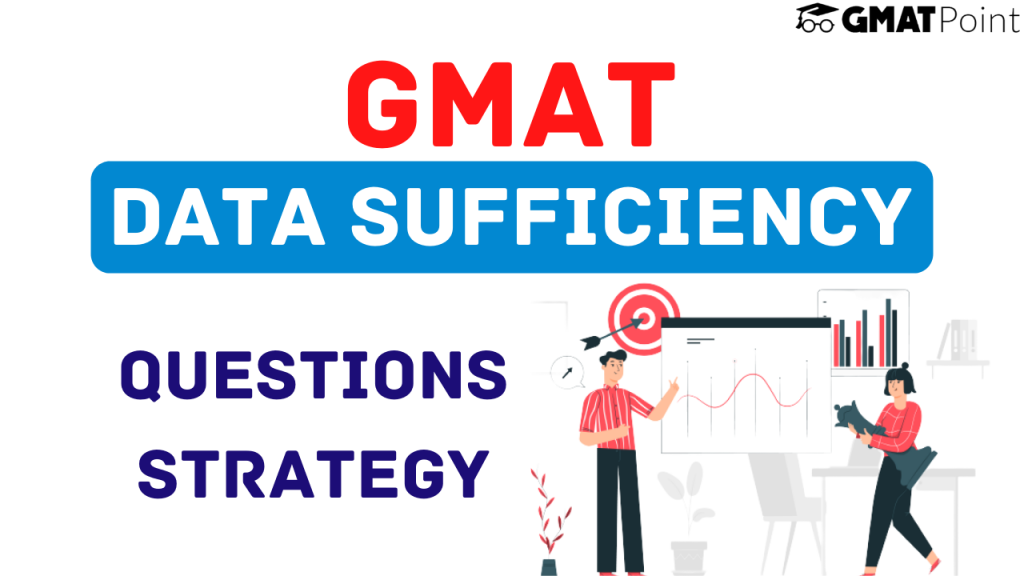# GMAT Data Sufficiency Questions Strategy# GMAT Data Sufficiency Questions Strategy

Data Sufficiency questions constitute a major chunk of the Quant section of the GMAT. Of the 31 questions that appear in this section, you can expect close to 50% of the questions from data sufficiency. In this article, we will be looking into –

1. What are GMAT Data Sufficiency Questions?
2. Why are GMAT Data Sufficiency Questions considered tricky?
3. A proper GMAT Data Sufficiency Questions Strategy
4. Tips to keep in mind

## What are GMAT Data Sufficiency Questions?

Data Sufficiency or DS questions are questions that have a question with partial information followed by two statements in the form of:

Statement 1: …….

Statement 2: ……..

These two statements will be followed by five options.

a) Statement (1) ALONE is sufficient, but Statement (2) alone is not sufficient
b) Statement (2) ALONE is sufficient, but Statement (1) alone is not sufficient
c) Both Statements (1) and (2) TOGETHER are sufficient, but NEITHER statement ALONE is sufficient.
d) EACH statement ALONE is sufficient
e) Statements (1) and (2) TOGETHER are NOT sufficient

These options are the same for every Data Sufficiency Question and it is highly advisable that you get used to them.

In Data Sufficiency Questions & Answers, you do not need to solve the question to get an answer. You only need to find out how much information is needed to find out the answer.

For example – If a question asks you the value of a variable x, and you can easily say that if you combine the two statements, you can reach the answer, you do not need to go ahead and find out the actual answer. You can simply mark Option C and carry on.

## Why are GMAT Data Sufficiency Questions & Answers considered tricky?

• First, we are inherently taught how to solve MCQ questions, but not taught how to solve Data Sufficiency questions. It takes some time to get used to them.
• We tend to assume some information that has not been explicitly stated, and end up marking the wrong option.
• We do not follow a proper approach and tend to mix up the statements.
• We miss out on some information while combining the two statements and end up with an incorrect response.

## GMAT Data Sufficiency Questions Strategy

Before we get started with the strategy to solve Data Sufficiency questions, it is important to know that there is not a single strategy to solve these questions. There are multiple strategies that can be employed to solve these questions. Also, if you are using a particular strategy and getting things correct, do not experiment with new strategies. A strategy is meant to maximise the number of correct responses, and any strategy that does the same is a good strategy. If you haven’t yet come up with a strategy or your current strategy is not working out in your favour, here. we are sharing a general strategy that many test-takers tend to follow.

Add the information to the information given in the statement and try to find out if it is possible to get the value/answer asked in the question.

Step 2: If it is sufficient, do the same for the second statement. If this too is sufficient, mark option D or mark Option A/B.

Step 3: If none of the statements is individually sufficient, combine the two pieces of information and try to solve for the value/answer. If you get the value, you can mark C else E.

Note:

2. In each step, you can eliminate a few of the options and hence have a higher chance of getting the answer right even if you are taking a guess.

## Tips to keep in mind:

1. Try to invest more time in Data Sufficiency questions than in Problem Solving Questions.
2. Do not get stuck in a question for long. If you find yourself trapped in a question for long, take a calculated guess[based on eliminated options] and move on.